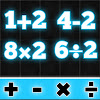# Math Quiz Game :Learn add subtract multiply Divide

1.0 by App Mantri

Math Quiz Game is a very simple.

There are Four type of feature that you can use to earn coin easily

Subtract Question(subtracting two number base question)

Multiply Question(multiplying two number base question)

Divide Question(Divide two number base question)

For every Correct Answer You will get Coin and every Incorrect Answer Coin will reduced

Easy To Use
No Personal Information Need To Use This App.

Safe And Secure No Data Need No Special Permission Need

###### Package Name
com.appmantri.mathquizgame
Educational
###### Update Date
September 12, 2021
5.2M